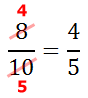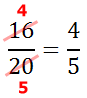Reducing Fractions
 Home > Lessons > Reducing Fractions Search | Updated December 21st, 2017
Introduction

This lesson will inform you how to reducing fractions. Here are the sections within this lesson page:

 Prerequisite Knowledge To simplify fractions it is important to know how to determine factors of a number. It is strongly recommended that you quickly (or not so quickly) review our divisibility lesson before progressing with the following sections.esson: Divisibility Check Ratios are Everywhere We use ratios all the time. When we look at pieces of art, we compare lengths to widths. If we buy something in a foreign land, we use ratios to convert the amount into a familiar currency. To travel, we use maps, which are scaled down versions of roads.     These are all examples of ratios and ratios often need to be reduced to be most meaningful.     The examples that follow will demonstrate how to reduce fractions. Example 1To reduce this fraction, we divide the numerator and the denominator by the same amount. We chose a common factor to do this.    The numerator and denominator have a common factor: 2. The division looks like this.Of course, 8 and 10 also have a common factor, which is again 2.We could have reduced this original problem faster had we noticed the larger common factor, which is 4.4/5 is the completely reduced solution.ideo: Reducing Fractionsuiz: Reducing Fractions Example 2The numerator and the denominator have several factors in common. But, let us start with the most easily recognized. Since the numerator and denominator are both even, they are divisible by 2.It can be seen that the numerator and denominator are both divisible by 3. So, let us divide the numerator and the denominator by 3.This is the fraction that is completely reduced.ideo: Reducing Fractionsuiz: Reducing Fractions Instructional Videos Use this instructional video to learn how to reduce fractions.ideo: Reducing Fractions Interactive Quizzes Use this interactive quiz to determine if you fully comprehend the sections above.uiz: Reducing Fractions Related Lessons Try these lessons, which are related to the sections above.esson: Multiplying Fractionsesson: Dividing Fractionsesson: Fractions, Decimals, Percentsesson: Proportionsesson: Adding and Subtracting Fractionsesson: Similar Figuresesson: Dilations